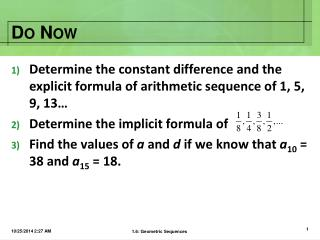Download PresentationDo Now

Loading in 2 Seconds...

# Do Now - PowerPoint PPT Presentation

Do Now. Determine the constant difference and the explicit formula of arithmetic sequence of 1, 5, 9, 13… Determine the implicit formula of Find the values of a and d if we know that a 10 = 38 and a 15 = 18. Worksheet Key. Geometric Sequences. section 12.3. Definitions.I am the owner, or an agent authorized to act on behalf of the owner, of the copyrighted work described.
Download Presentation## Do Now

An Image/Link below is provided (as is) to download presentation

Download Policy: Content on the Website is provided to you AS IS for your information and personal use and may not be sold / licensed / shared on other websites without getting consent from its author.While downloading, if for some reason you are not able to download a presentation, the publisher may have deleted the file from their server.

- - - - - - - - - - - - - - - - - - - - - - - - - - E N D - - - - - - - - - - - - - - - - - - - - - - - - - -
Presentation Transcript
1. Do Now • Determine the constant difference and the explicit formula of arithmetic sequence of 1, 5, 9, 13… • Determine the implicit formula of • Find the values of a and d if we know that a10 = 38 and a15 = 18. 1.6: Geometric Sequences

2. Worksheet Key 1.6: Geometric Sequences

3. Geometric Sequences section 12.3 1.6: Geometric Sequences

4. Definitions • A geometric sequence is a list of numbers that have a COMMON RATIO (r) meaning you DIVIDED by the same number. • To find r = DIVIDE THE SECOND NUMBER TO THE FIRST NUMBER 1.6: Geometric Sequences

5. Is it Geometric? Determine whether these sequences are geometric. If it is, determine the ratio. • {1, 3, 9, 27, 81...} • {180, 90, 0, –90, –180...} • {5, 1, 0.2, 0.04, 0.008...} • {–50, –32, –18, –8, –2...} YES r = 3 Arithmetic YES r = 0.2 NO 1.6: Geometric Sequences

6. Formulas Explicit Formula: Recursive Formula: 1.6: Geometric Sequences

7. Steps • Find the common ratio • Use the formula and determine the rule • Evaluate for the missing variable • Check 1.6: Geometric Sequences

8. Example 1 Find the 6th term and explicit rule for given, a1 = 5, r = 2 Step 1: Find the common ratio. 1.6: Geometric Sequences

9. Example 1 Find the 6th term and explicit rule for given, a1 = 5, r = 2 Step 2: Use the formula and determine the term 1.6: Geometric Sequences

10. Example 1 Find the 6th term and explicit rule for given, a1 = 5, r = 2 Step 3: Evaluate for the missing variable 1.6: Geometric Sequences

11. Example 1 Find the 6th term and explicit rule for given, a1 = 5, r = 2 Step 4: Check 1.6: Geometric Sequences

12. Example 2 Find the 10th term and explicit rule for the following geometric sequence of 16, –8, 4, –2,… 1.6: Geometric Sequences

13. Your Turn Find the 5th term and explicit rule for given a1 = 1/6, a2 = –1/18. Leave answers in fraction form. 1.6: Geometric Sequences

14. Example 3 Find the 8th term of the geometric sequence with a3 = 36 and a5 = 324. Step 1: Find the common ratio. 1.6: Geometric Sequences

15. Example 3 Find the 8th term of the geometric sequence with a3 = 36 and a5 = 324. Step 2: Find a1. Consider both the positive and negative values for r. or 1.6: Geometric Sequences

16. Example 3 Find the 8th term of the geometric sequence with a3 = 36 and a5 = 324. Step 3: Write the rule and evaluate for a8. Consider both the positive and negative values for r. or 1.6: Geometric Sequences

17. Example 4 Find the 8th term of the geometric sequence with a2 = 80 and a4 = 1280 1.6: Geometric Sequences

18. Your Turn Find the 8th term of the geometric sequence and its implicit formula with a3 = 108 and a5 = 3888 1.6: Geometric Sequences

19. Assignment Geometric Sequences Worksheet 1.6: Geometric Sequences